### IMO Shortlist 2014 problem G5

Kvaliteta:
Avg: 0,0
Težina:
Avg: 8,0

Let$ABCD$ be a convex quadrilateral with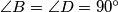$\angle B = \angle D = 90^\circ$. Point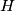$H$ is the foot of the perpendicular from$A$ to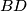$BD$. The points$S$ and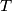$T$ are chosen on the sides$AB$ and$AD$, respectively, in such a way that$H$ lies inside triangle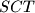$SCT$ and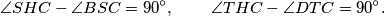Prove that the circumcircle of triangle SHT is tangent to the line$BD$.

(Iran)

Izvor: https://www.imo-official.org/problems/IMO2014SL.pdf